[ HOME ]   [ NEXT ]   [ BACK ]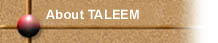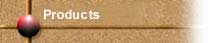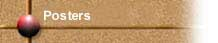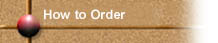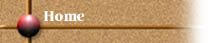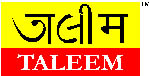A       D I V I S I O N      O F      E K L A V Y A      E D U C A T I O N      F O U N D A T I O N

 Code : TLM-M-001 Age : 5 - 7 yrs. Name: Place Value Cards -1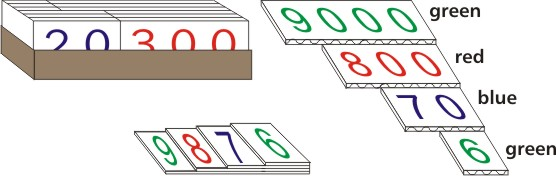Each set contains    Cards numbered         1    -       9 (green)       10   -      90 (blue)     100  -     900 (red)   1000  -   9000 (green)
 How to use

 This material can be used to demonstrate the concept of the place value of a number. A single student can use this material. This material helps children to visualise the place value of a number. The size of the card increases as the place value increases. Step 1: The student takes a fresh sheet of paper, draws a margin, writes her name and date on the top right hand corner and the name of the exercise in the center of the page. Step 2: Then she writes a four digit number in the paper, say 9876. Step 3: She then checks the left most digit in the number. The left most digit in the given example being 9, she checks for the card whose left most digit is 9 and the number of digits in the card are 4. She finds that the card is 9000. She then places the card on the table. Step 4: She then checks the second digit from left in the number. The second digit being 8, she again checks for the card whose left most digit is 8 and the number of digits in the card are 3. She finds that the card is 800. She then places this card on top of the earlier card, taking care that the digit 9 is visible. Step 5: She then checks the third digit from left in the number. The third digit being 7, she again checks for the card whose left most digit is 7 and the number of digits in the card are 2. She finds that the card is 70. She then places this card on top of the earlier two cards, taking care that the digit 9 and 8 are visible. Step 6: She then checks the fourth digit from left in the number. The fourth digit being 6. She again checks for the card whose left most digit is 6 and the number of digits in the card is 1. She finds that the card is 6. She then places this card on top of the earlier three cards, taking care that the digit 9, 8 and 7 are visible. Step 7: She now realises that 9876 is actually 9000 + 800 + 70 + 6 and as you move from left to right the place value of the number changes. In this case it is 1000, 100, 10 and 1and the face value of the number is 9876.

 Definition of Place Value

 Each digit in a number has a value according to its place in the number. This value is called its place value (e.g. In the number 55, the first 5 from left has a place value of 10, while the second 5 from left has a place value of 1. Hence the number 55 can be represented as 50 + 5).
 Principle

 Children are introduced to the concept of place value through words and symbols used to describe numbers The number 65, for instance, means six times 10 and five more. Similarly the number 222 means, 2 times hundred + 2 times 10 + 2 more.

 Teaching Learning Materials (TLM) can make learning much more effective and fun in classrooms. Taleem is an initiative in this direction.

[ HOME ]   [ NEXT ]   [ BACK ]